# cgTalk #04

### Fun with distance fields

Are we there yet?

• space for the next slide
• backspace for the previous slide
• up/down to navigate in current chapter

## Why?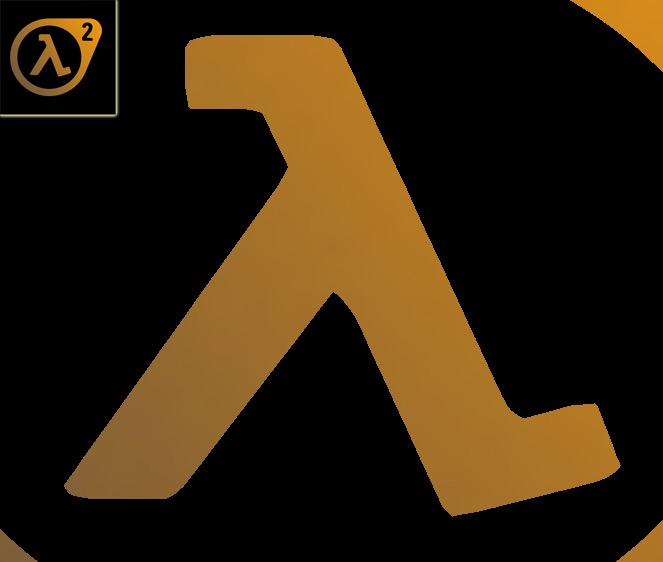source: valve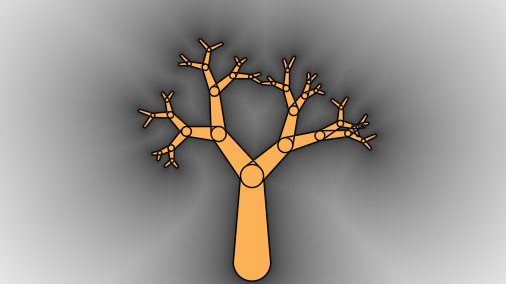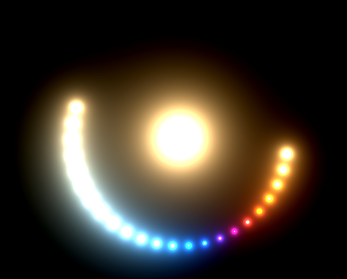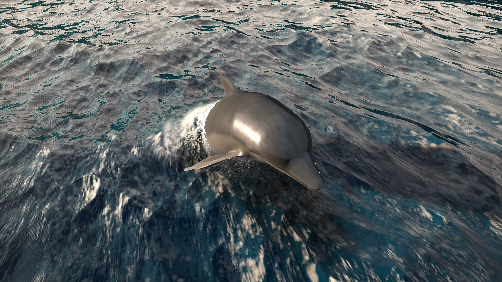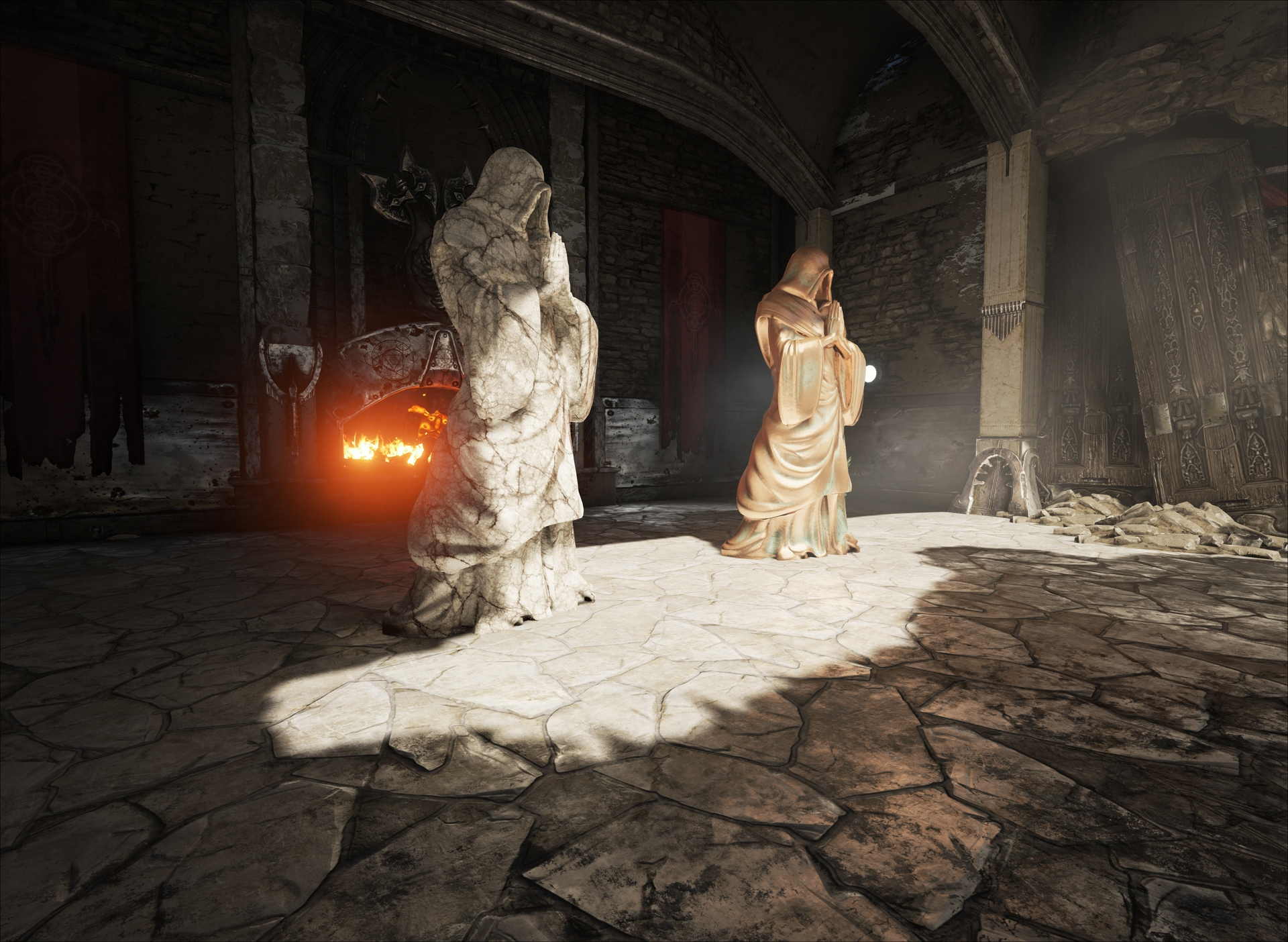source: Epic Games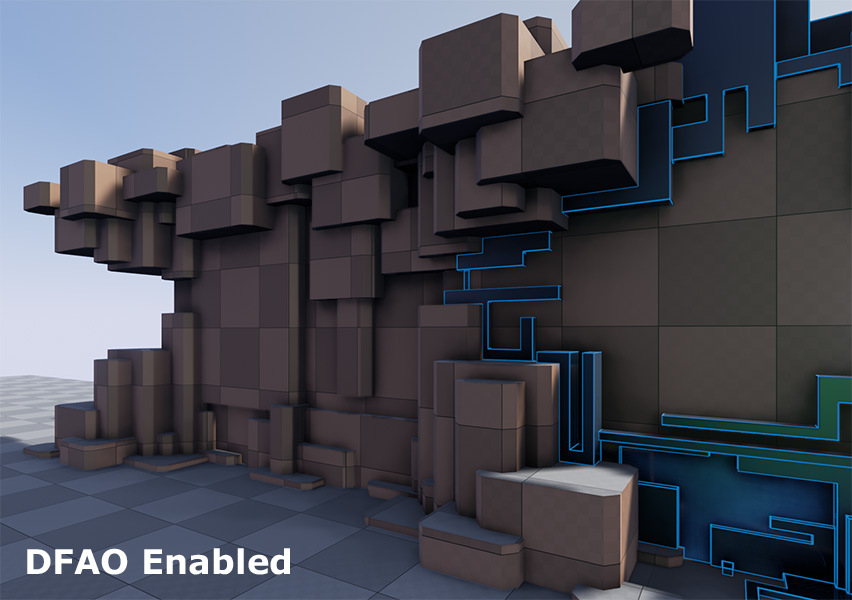www.tomlooman.com - UE4

## Agenda

• Distances
• 2D-Distance maps
• 2D-Distance functions
• 3D-Distance fields - functions
• 3D-Distance fields - geometries

## Distance

### Distance

What's distance?

• the property created by space between two points
• What's space? $~\rightarrow~\mathbb{R}^n \rightarrow \mathbb{R}^2, \mathbb{R}^3$

Distance functions dist(p, q)

• euclidean distance:$~\textbf{dist}(p, q) = \sqrt{\sum_{i=0}^{n}{(p_i - q_i)^2}}$
• manhattan distance:$~\textbf{dist}(p, q) = \sum_{i=0}^{n}{\lvert p_i - q_i\rvert}$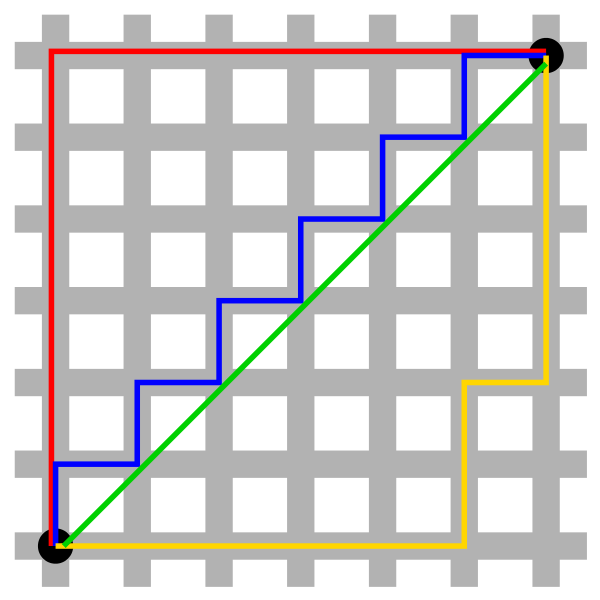source: wiki

### Distance fields

Distances (distance field values) can be obtained by

• sampling distance field maps
• greyscale, rgba-encoded, ...
• great for complex patterns (glyphs, ...)
• evaluating distance functions
• euclidean, manhattan, ...
• no sampling!
• only trivial "objects"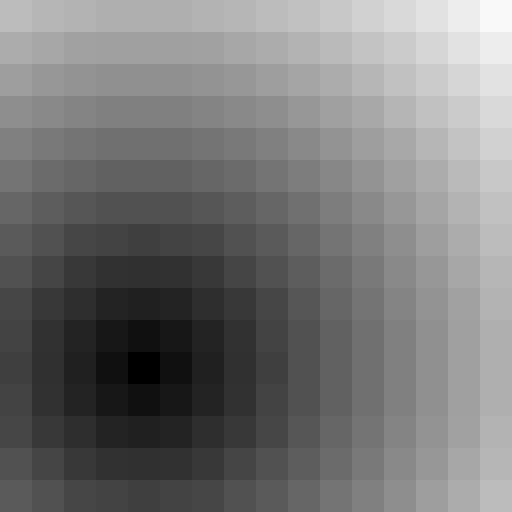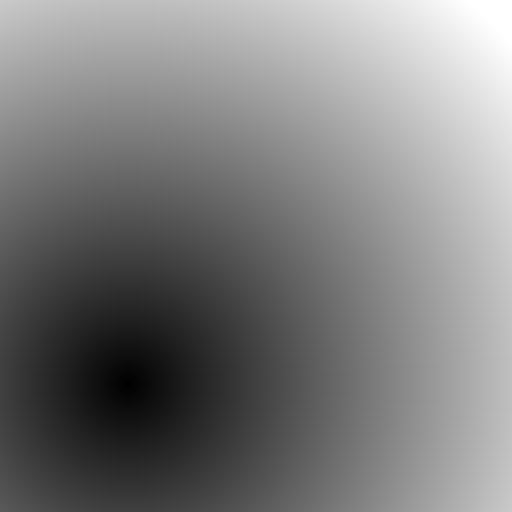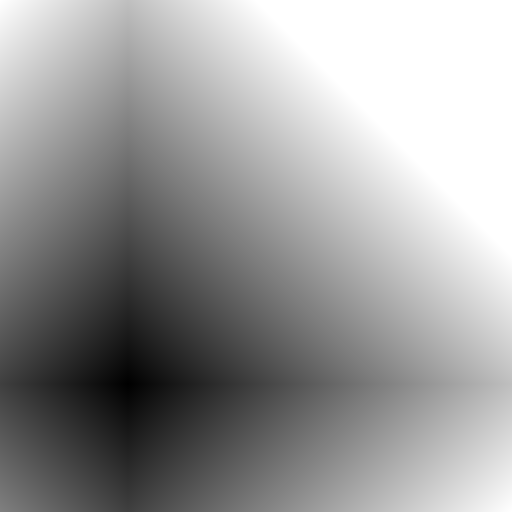### Using distances

Input

• 2D: texture Coordinate, 2D-coordinate on surface, ...
• 3D: generated 3D (camera)-ray, ...

Output:

• boolean: use mix to blend the "in"-color:
colRes = mix(colIn, colRes, step(0.0, distance));
• use fwidth to calculate fade-width
• blend between 0-1 using smoothstep
colRes = mix(colIn, colRes, smoothstep(0.0, fwidth(distance), distance));
• bonus: widen the falloff for a glow
colRes = mix(colIn, colRes, smoothstep(0.0, glowDistance, distance));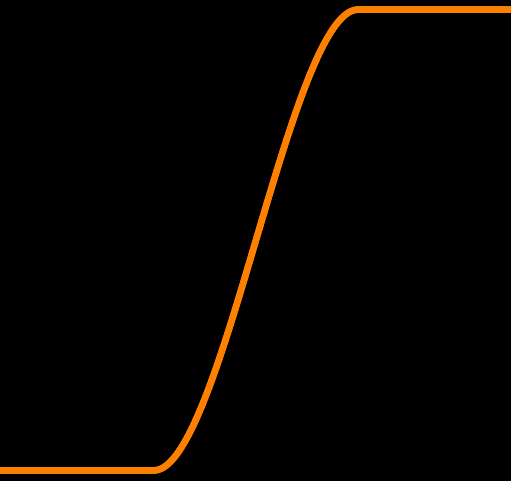smoothstep

## 2D-Distance maps

### SDF - Signed Distance Field

• encode distance to the other side using signs
• 0, distance to 1: $\rightarrow <0$
• 1, distance to 0: $\rightarrow >0$
• shift distance into "single-channel" range:
• $<0 \rightarrow [0; 0.5)$
• $>0 \rightarrow (0.5; 1.0]$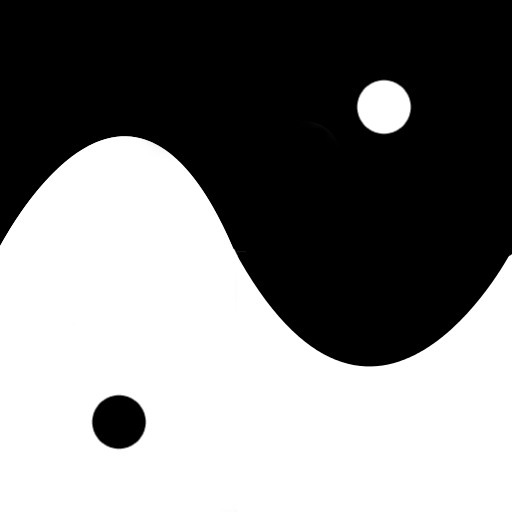original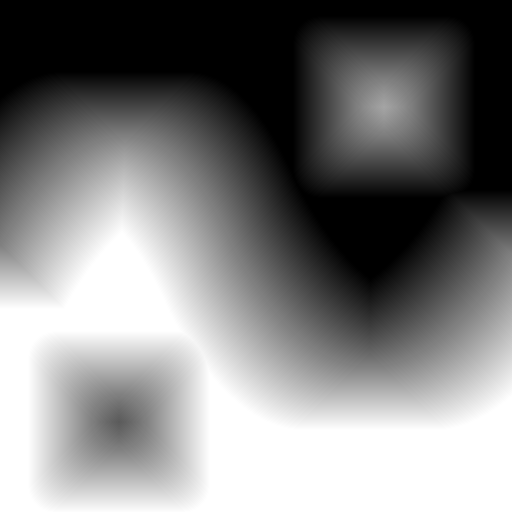sdf

### Using SDF 1/3

1. load small sdf-map with bilinear filter!
2. sample distance field texture
3. use 0.5 as an edge between color A and color B
4. throw a fwidth in the mix

      uniform sampler2D u_SdfMap;

varying vec4 v_Color;
varying vec2 v_TexCoord;

void main() {
float distance = texture2D(u_SdfMap, v_TexCoord).a;
float width = fwidth(distance);
float alpha = smoothstep(0.5 - width, 0.5 + width, distance) * v_Color.a;
gl_FragColor = vec4(v_Color.rgb, alpha);
}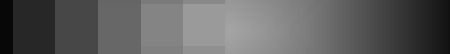nearest | bilinear

### Using SDF 2/3

• small sdf-map allows for large rendering
• fast: single HW-based filtered value + minimal computation
• dynamic thickness: change the edge-valuefrom 64x64

source: valve320x320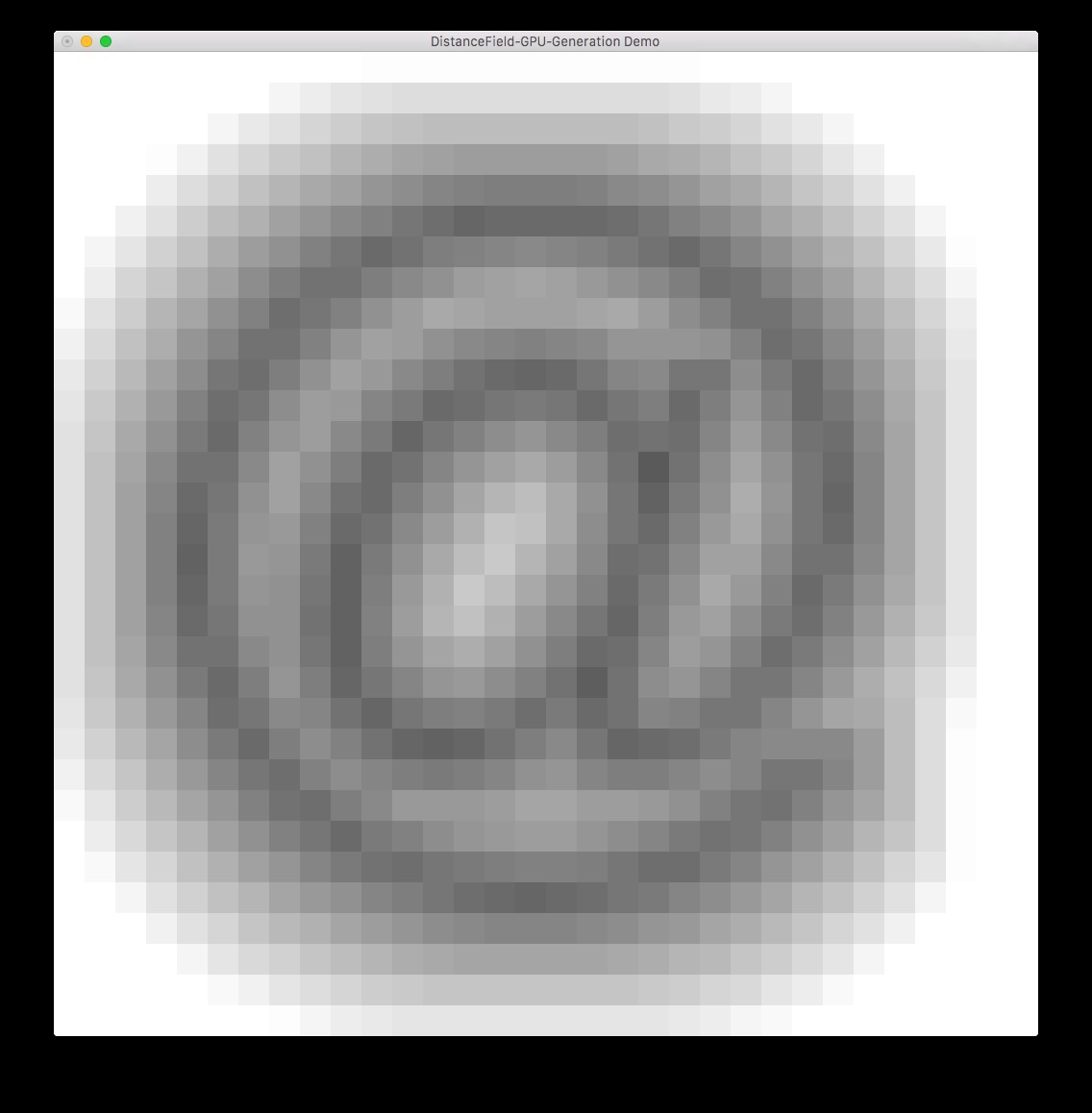sdf:32x32

### Using SDF 3/3

Problems with SDF's

• fine structures are lost in SDF sampling
• bilinear interpolation can be recognized (see here)
• multiple bit-planes not always combinable (see here)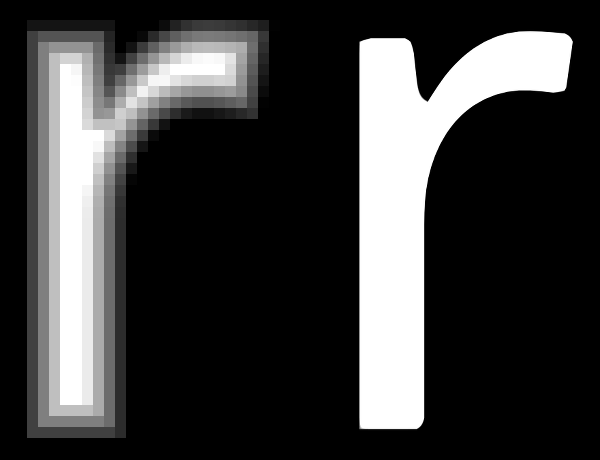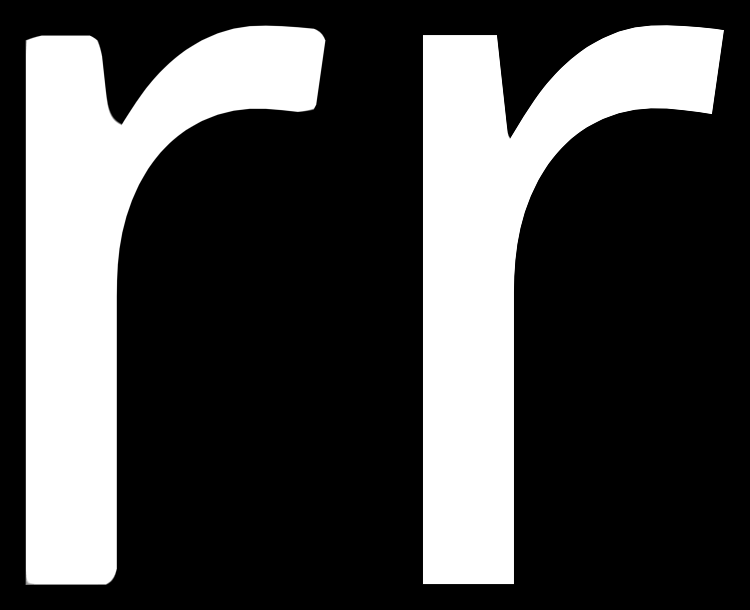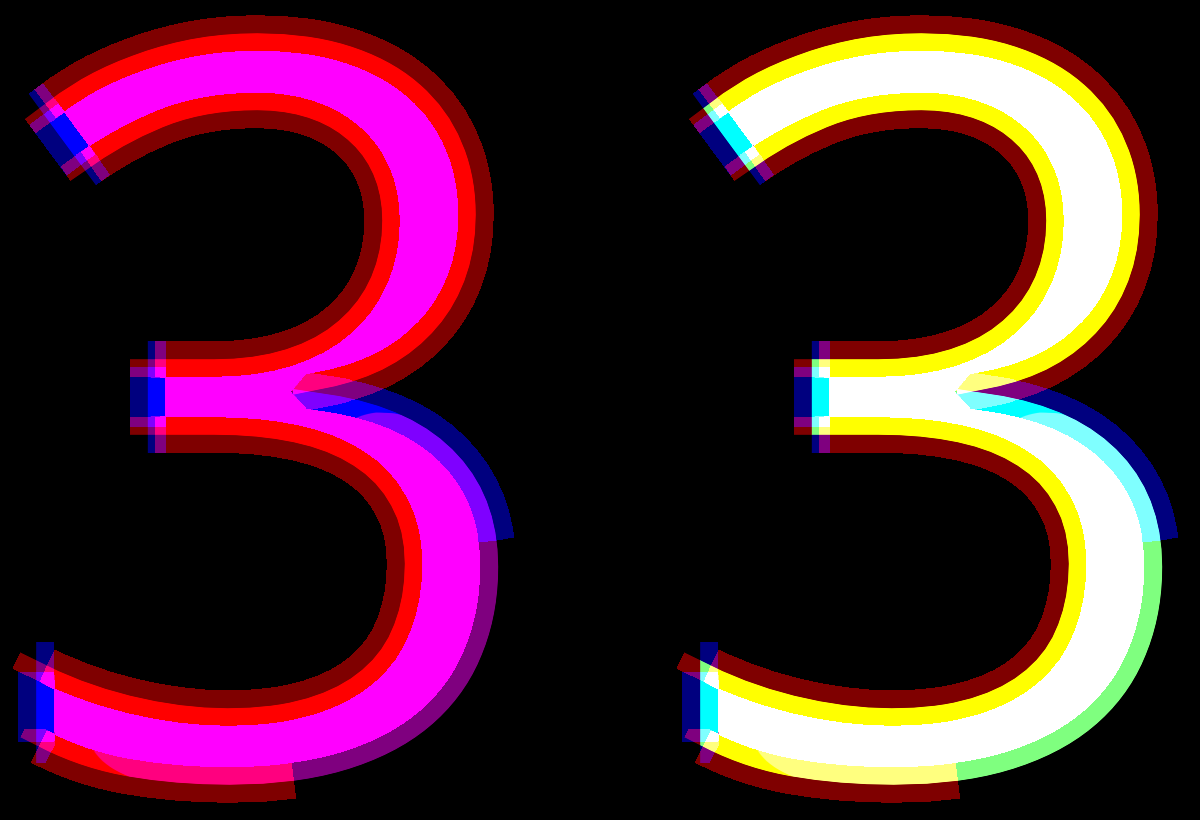source: lambdacube3d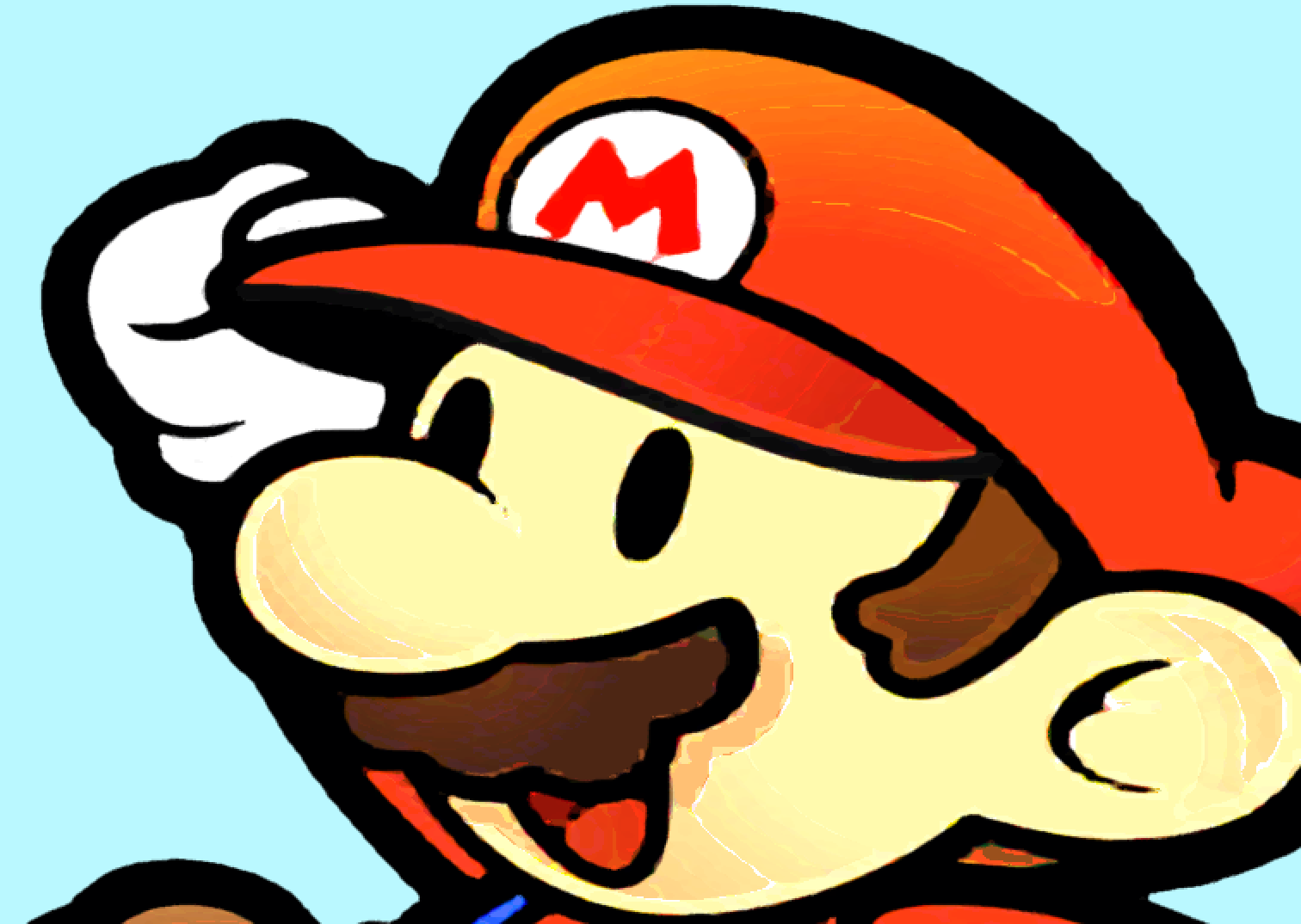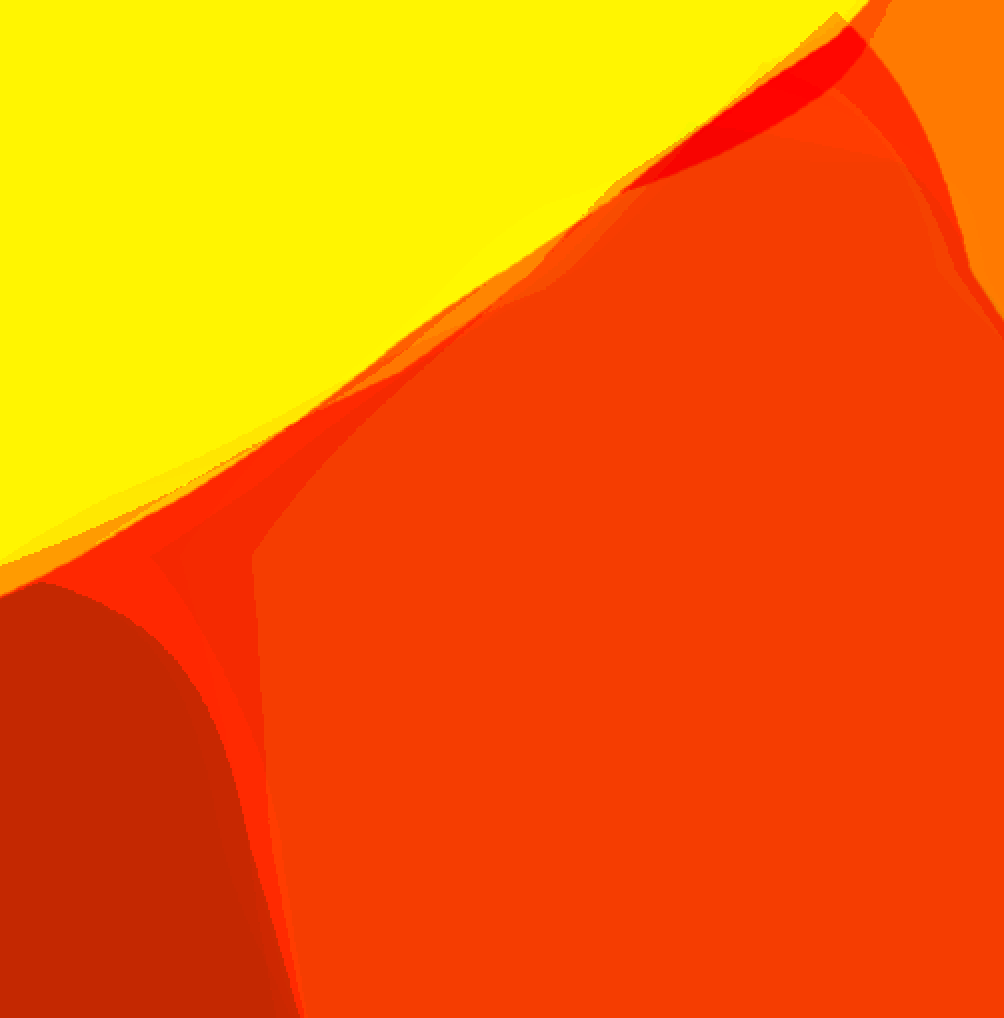source: gpuhacks

### Generating a SDF

Basic idea:

1. Input:
• large texture (e.g. $4096^2$)
• target output size (e.g. $64^2$)
• spread for effect (e.g. $32$)
2. Calculate distance field for target texture:
• for every texel calc corresponding in/out value
• calculate distance to nearest "other" texel
3. map distance into target-range

Note: there are more efficient algorithms out there.

### Generating a SDF on the GPU 1/2

Now let's use some shaders for that :-)

• use ping-pong Render-To-Texture: A $\rightarrow$ B $\rightarrow$ A
• Filter original-sized images with simple GLSL Image-Filters:
1. Store marked bit & target vector to other side
2. Detect edges in source image: mark it, target=(0/0)
3. Iterative PP-RTT until whole texture marked:
• For every pixel of current texture
• if (not marked) & any(neighbour $n$ marked):
• mark current
• target = min($n$.target + ($n$ - current))
4. Convert target vector to distance (max distance = $s\sqrt{2}$)
5. Render texture into final texture for target resolution

### Generating a SDF on the GPU 2/2input image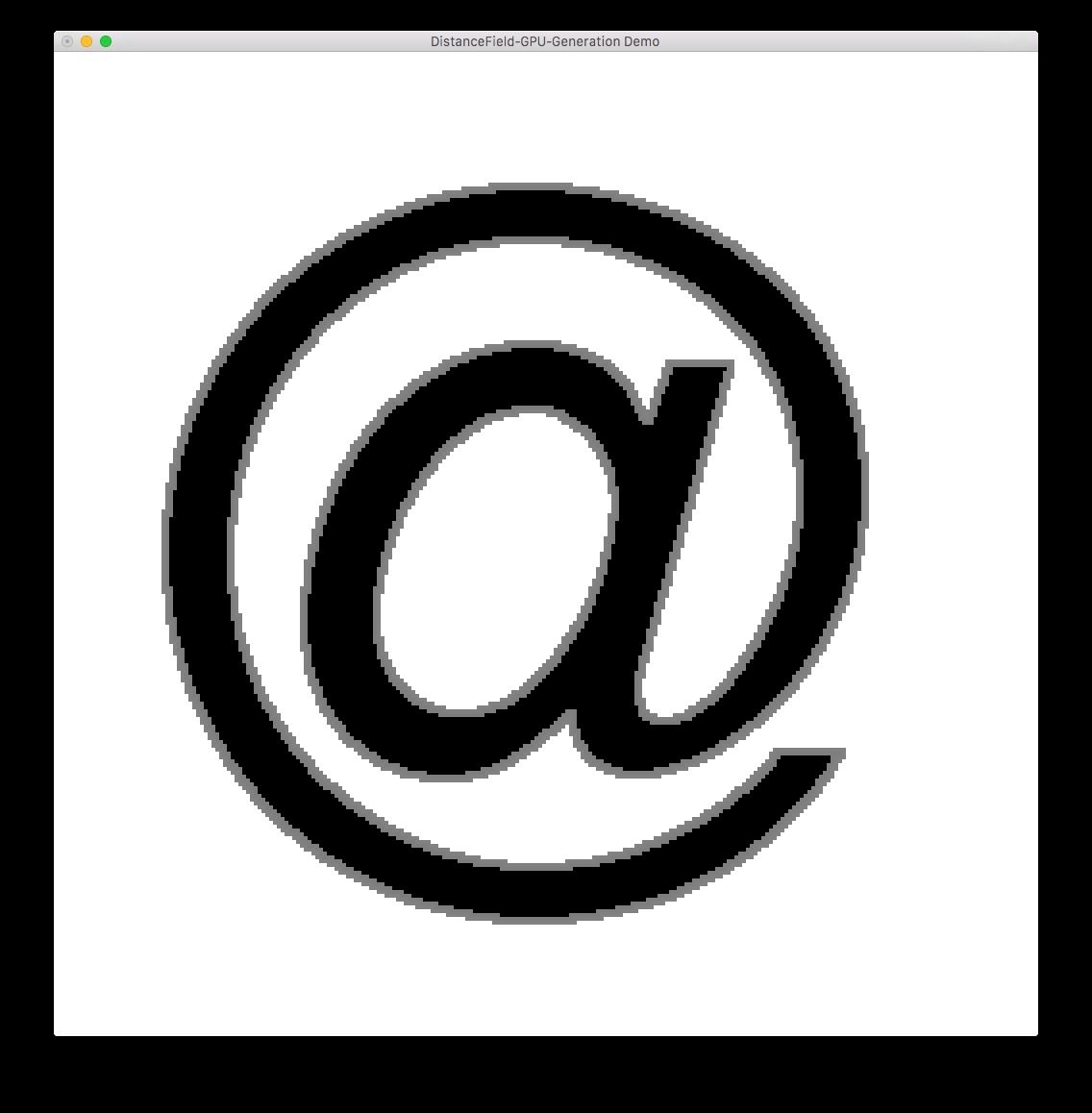init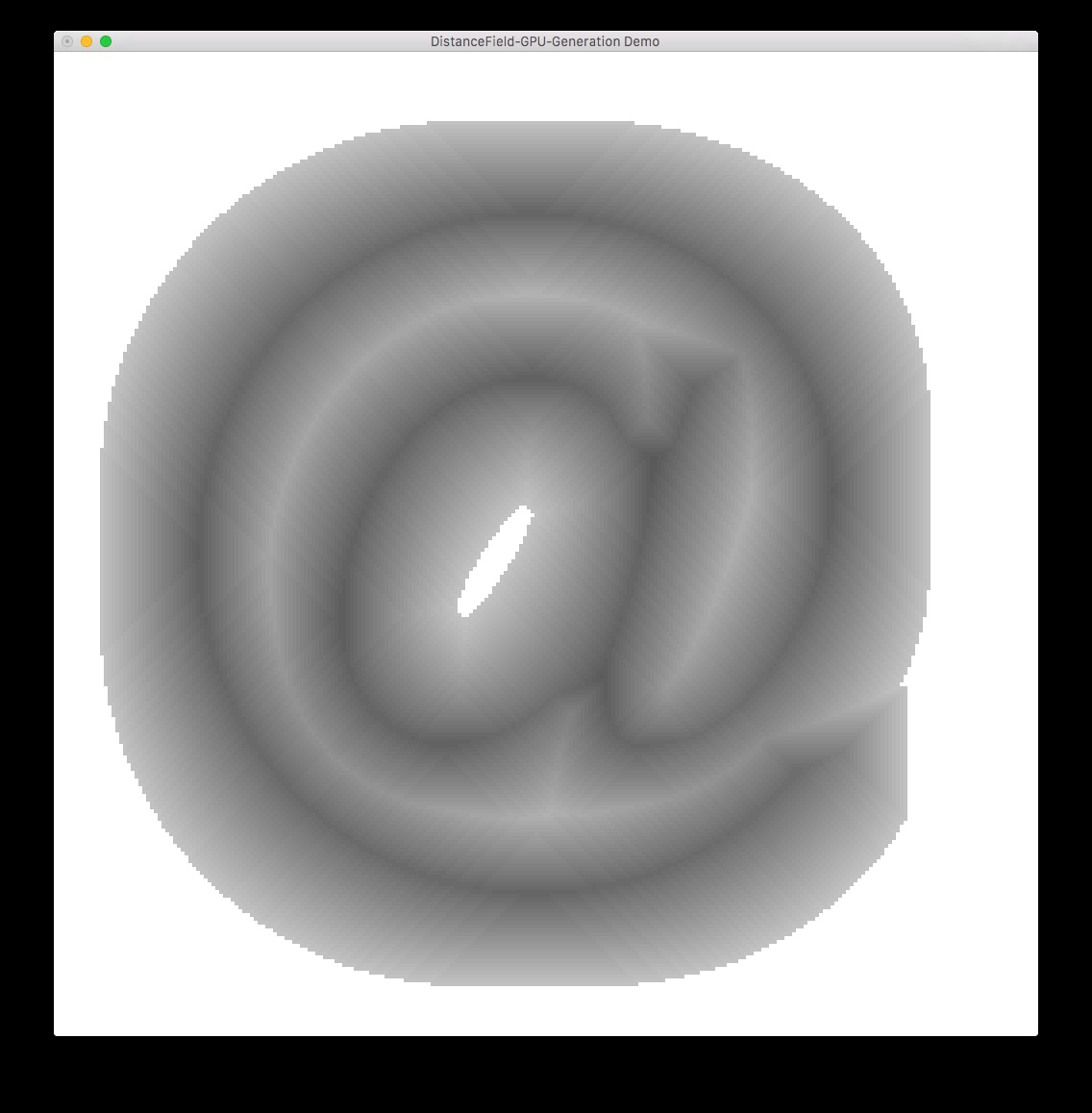step 16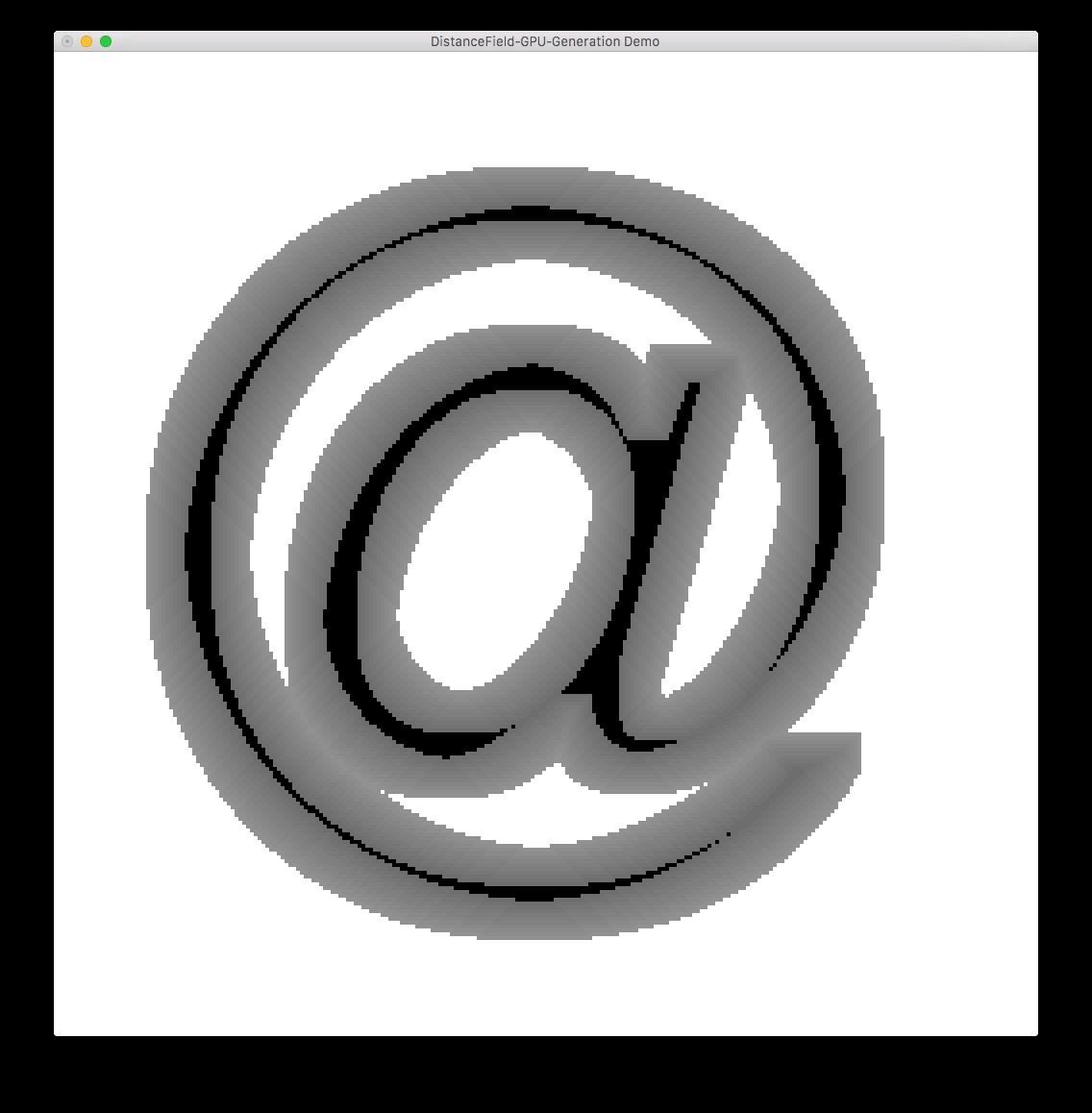step 4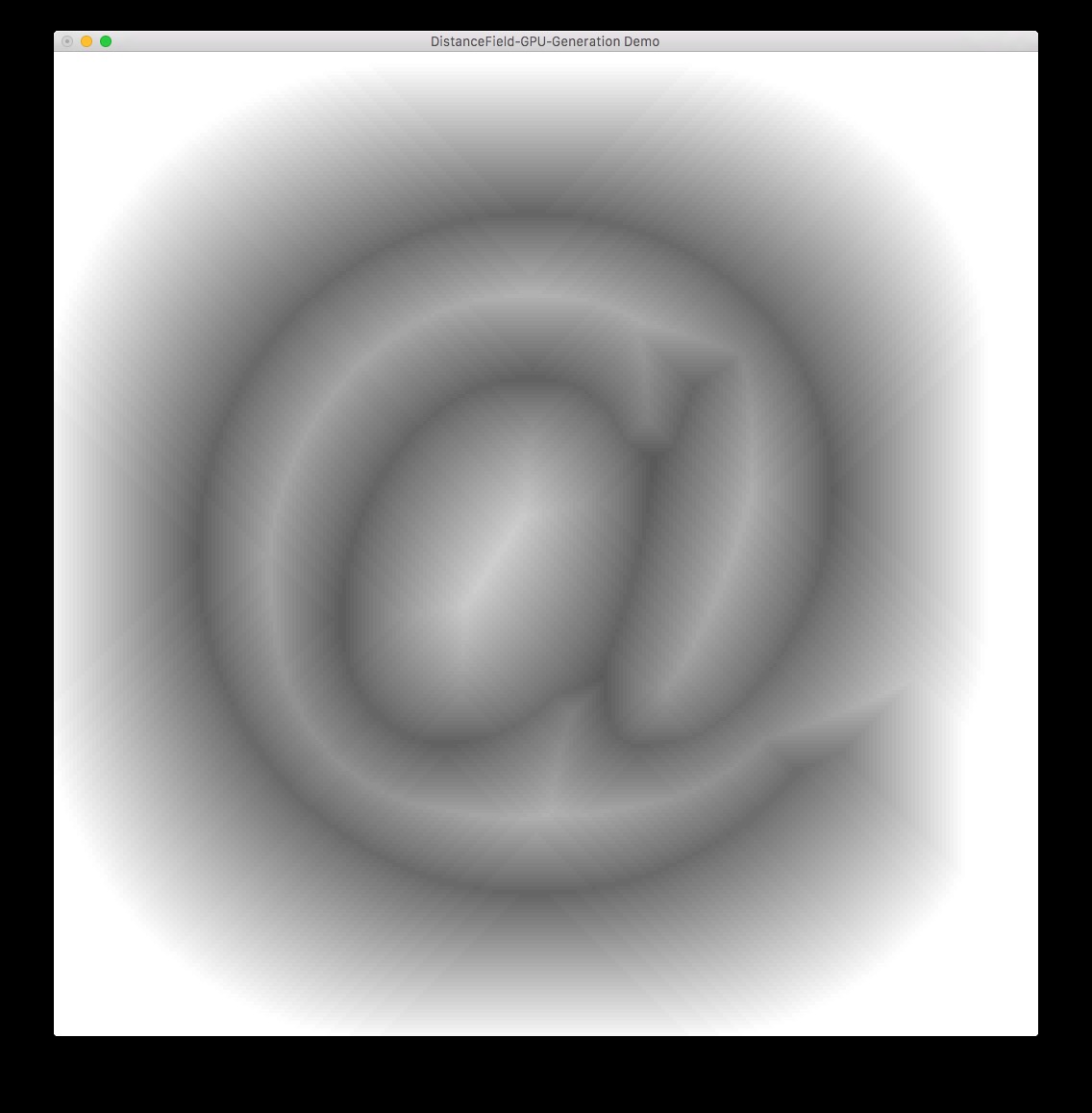step 32output image

## 2D-Distance functions

### 2D-Distance functions

Alternative to distance maps: evaluate a distance function!

• For certain primitives a function is easy:
circle(pos, center, rad)  = length(pos - center) - rad;
diamond(pos, center, rad) = dot(abs(pos - center), vec2(1.0)) - rad;
• Even complex shapes can be realized: Antialiased 2D Grid, Marker, and Arrow Shaders
• works great with fragment derivative antialiasing!
• functions can be combined (think CSG!) and repeated
union(dist1, dist2)  = min(dist1, dist2);
subtract(dist1, dist2) = max(-dist1, dist2);
intersect(dist1 = dist2) = max(dist1, dist2);
• the coordinate system can be transformed

### Simple 2D-Distance functions 1/2

circle(pos, center, rad)  = sqrt(pow(pos - center, 2.0)) - rad;
diamond(pos, center, rad) = dot(abs(pos - center), vec2(1.0)) - rad;

### Simple 2D-Distance functions 2/2

union(dist1, dist2)  = min(dist1, dist2);
subtract(dist1, dist2) = max(-dist1, dist2);
intersect(dist1 = dist2) = max(dist1, dist2);
rotate(coord) = mat2(cos(ang), -sin(ang), sin(ang), cos(ang)) * coord;

### 2D Metaballs

• Metaballs are organic looking objects, that can "stick" together.
• Each ball is defined through a distance function $f$: $$f(x, y, z) = \frac{1}{(x - x_0)^2 + (y - y_0)^2 + (z - z_0)^2}$$
• The distance of multiple metaballs is added together: $$balls(x, y, z) = \sum_{i=0}^{n}f_i(x, y, z)$$
• If the summed distance is bigger than a threshold, the metaball is depicted solid.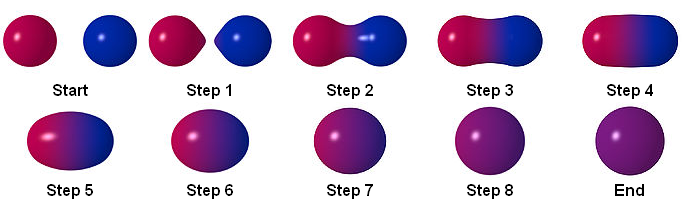source: wiki

### Animating 2D-Distance functions

Let's see what we can do with the following setup:

• distance functions: circle + x-axis-parallel rectangle
• screen segmented into 2d-grid of cells
• use time for animation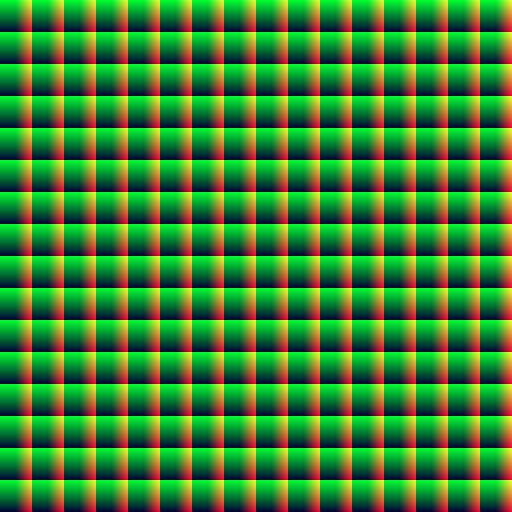Hint: We could move something circle-like along some cells, possibly highlighted by a rect!

### Complex example: 2D-Distance functions

Slightly different scenario:

• Keep the circle.
• Add a distance function for a heart. (tad complicated, see Antialiased 2D Grid, Marker, and Arrow Shaders....)
vec3 drawQuad(vec3 col, vec2 coord,
vec2 p1, vec2 p2, vec2 p3, vec2 p4,
float thickness, vec3 oldColor)
{
float sideA = sign(cross2D(coord - p1, p2 - p1));
float sideB = sign(cross2D(coord - p2, p3 - p2));
float sideC = sign(cross2D(coord - p3, p4 - p3));
float sideD = sign(cross2D(coord - p4, p1 - p4));
float dst = abs(sideA - sideB) + abs(sideB - sideC)
+ abs(sideC - sideD) + abs(sideD - sideA);
dst = clamp(dst, 0.0, 1.0);

vec3 res = mix(col, oldColor, dst);
res = drawLine(col, coord, p1, p2, thickness, res);
res = drawLine(col, coord, p2, p3, thickness, res);
res = drawLine(col, coord, p3, p4, thickness, res);
res = drawLine(col, coord, p4, p1, thickness, res);
return res;
}

### Complex example: 2D-Distance functions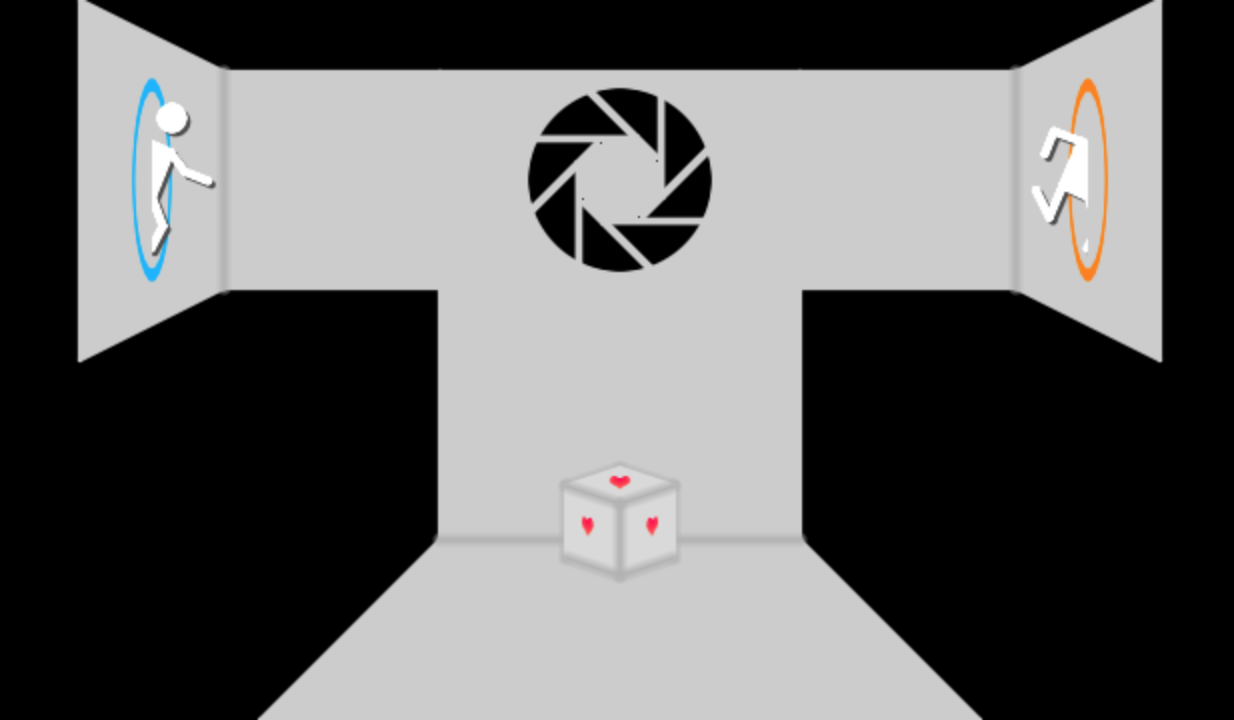## 3D-Distance fields

### (Recap) Raytracing

Raytracing is a rendering method

• Rays (from the camera) are traced through the scene: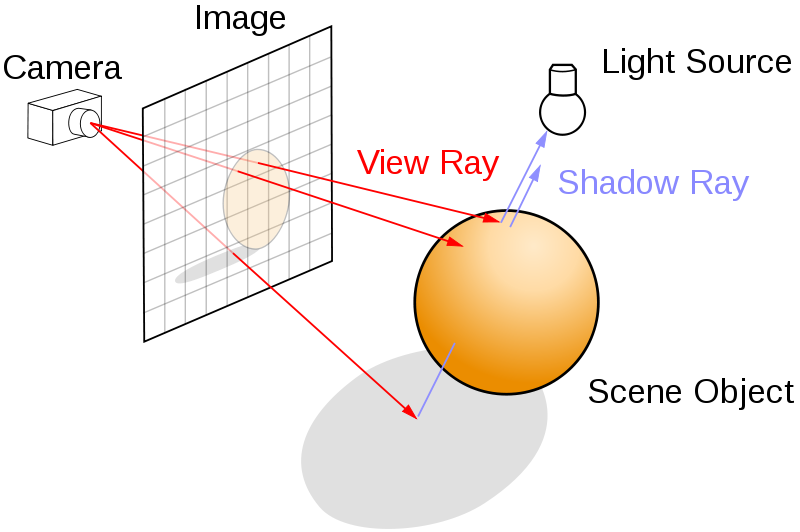source: wiki
• A view (or secondary, ...) ray is intersected with the scene
• Result is an exact, "singular" hit with "noise" artefacts

### Raymarching

Raymarching is related to raytracing

• Rays from the camera are marched through the scene: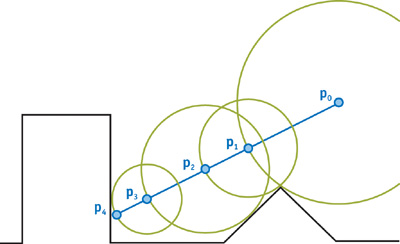source: GPU-Gems2
• At every step distances to all scene objects are evaluated
• The smallest distances leads to a new startpoint
• The march terminates if either
distance $<$ threshold || z-Limit || step limit

### Raymarching

r = generateRayForProjection(texCoord, fov);
while !(step-limit || z-limit)
d = getClosestDistanceWithScene
if (hitpoint < threshold)
r.origin += r.dir * d;
Potentially repeat above (shadow, reflection, AO, ...)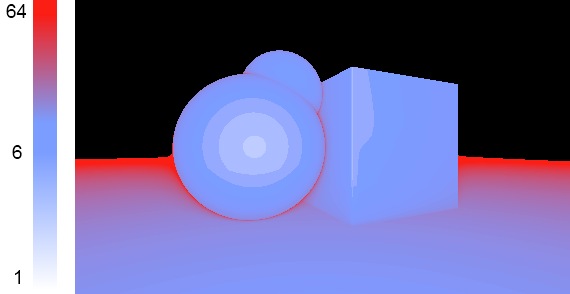iterations visualized

### Raymarching - Distance Functions

Great overview of distance functions here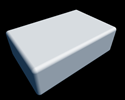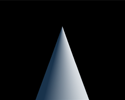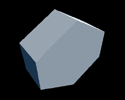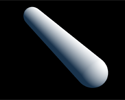• Distort/transform the input position for a model
• repeat the primitive position
• CSG (a, b):
• union: min(a, b)
• subtract: max(-a, b)
• intersect: max(a, b)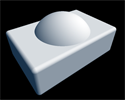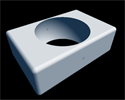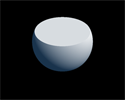### Raymarching - Scene

Here's a full, complex example on what can be done: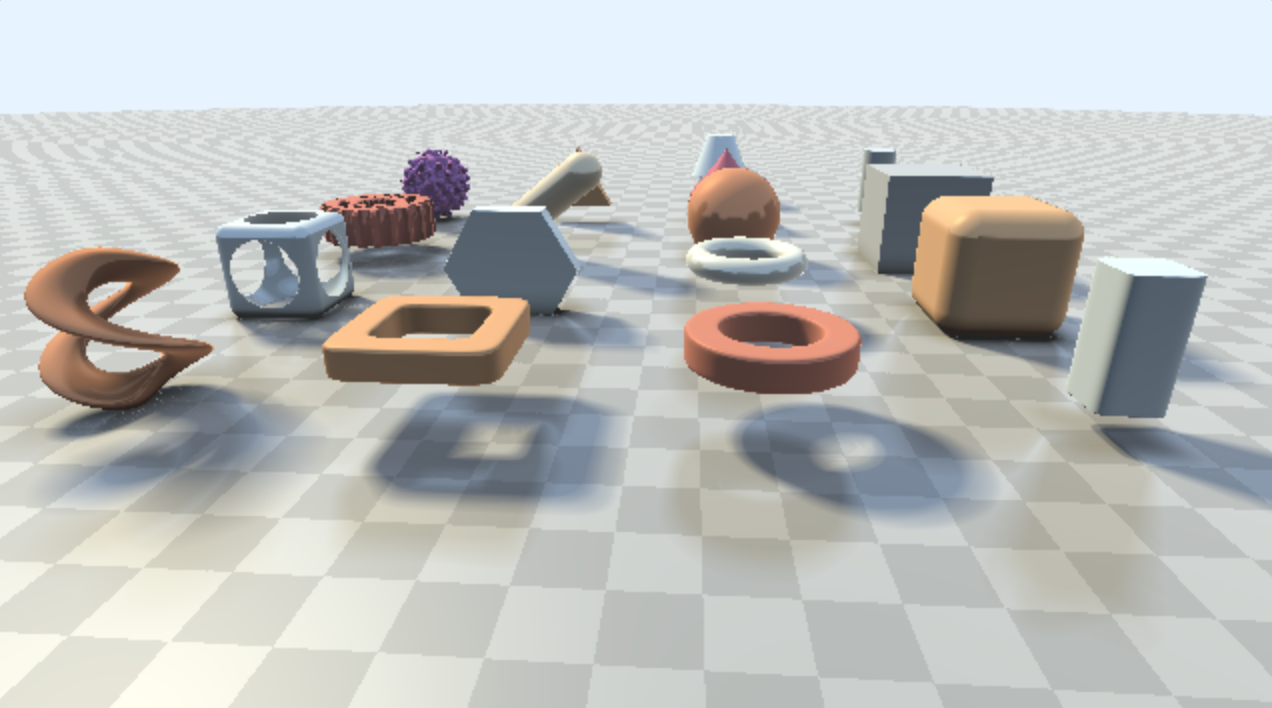### Raymarching - Illumination

Shading needs a normal: evaluate the scene around the hitpoint to obtain new "hitpoints" that form the normal.

• The amount of light that shines onto a surface determines if it's in shadow.
• This is determined by the visibility of the lights from a surfacepoint.
• Trace again: from light towards hitpoint:
• if you hit something else, hitpoint must be in shadow.
• Bonus: soft shadows (penumbra) are cheap :-)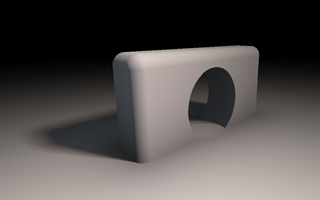### Raymarching - AO & reflection

Marching the scene from the hitpoint along the normal can be usefull for other Illumination effects:

• AmbientOcclusion is calculated by marching the scene around the normal
• Reflections can be calculated by marching the scene along the normal

## 3D-Distance fields*

### Raymarching - Geometries

In most real life applications we don't have many distance functions:

• we have polygonal geometries,
• where it's pretty darn hard to come up with distance functions!

In order to still use the benefits, we need to combine

• triangle-based geometries and
• distances for them

... something like the distance maps, but 3D...

enter.... *drummroll*

what?

### SVO - Sparse Voxel Octrees

Let's unroll it back-to-front:

• Octree: 3D-equivalent of a 2D-Quadtree of a 1D-binary tree.
• base: cube, that can be split along 3 axis in 8 sub-cubes
• Voxel: each node contains volumetric information:
• what's in that space, what's inside the cube?
• Sparse: the octree of voxels is sparsely populated. Easy :-).

### SVO - Sparse Voxel Octrees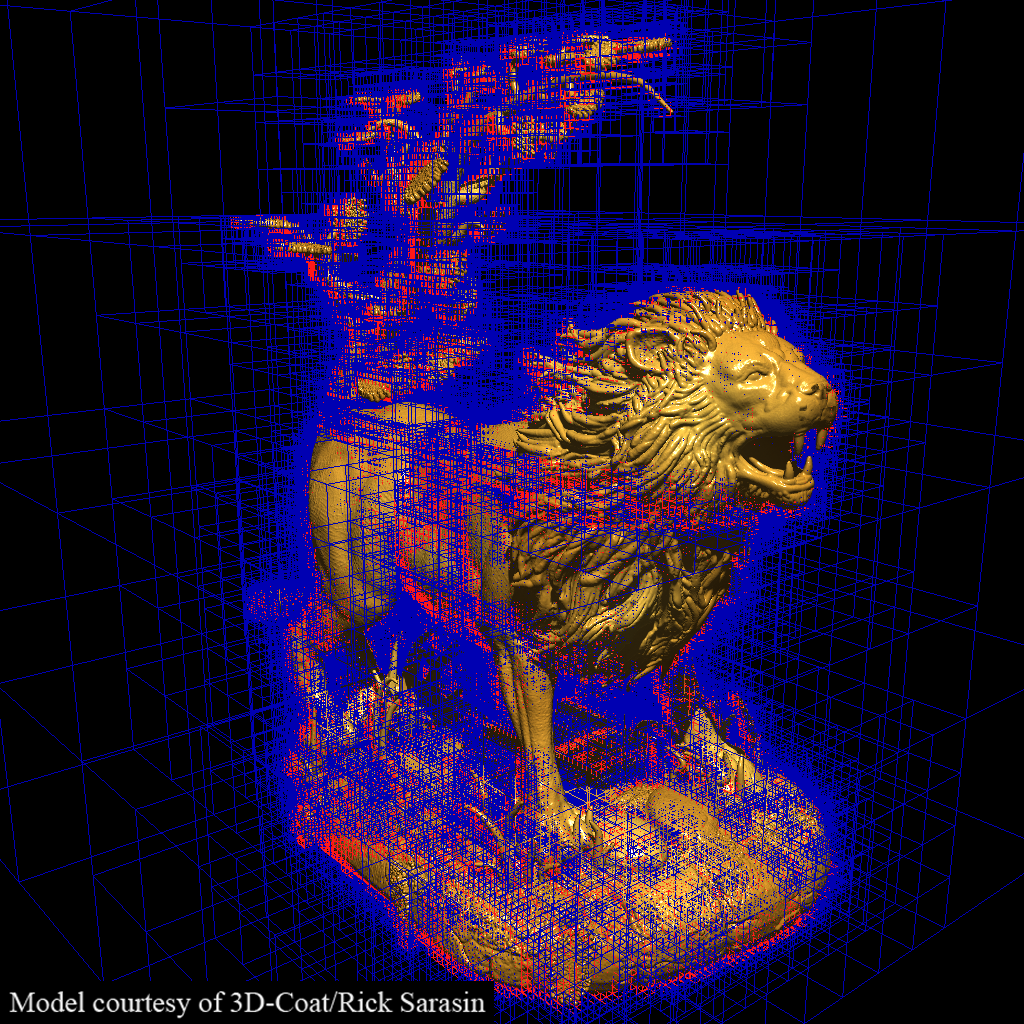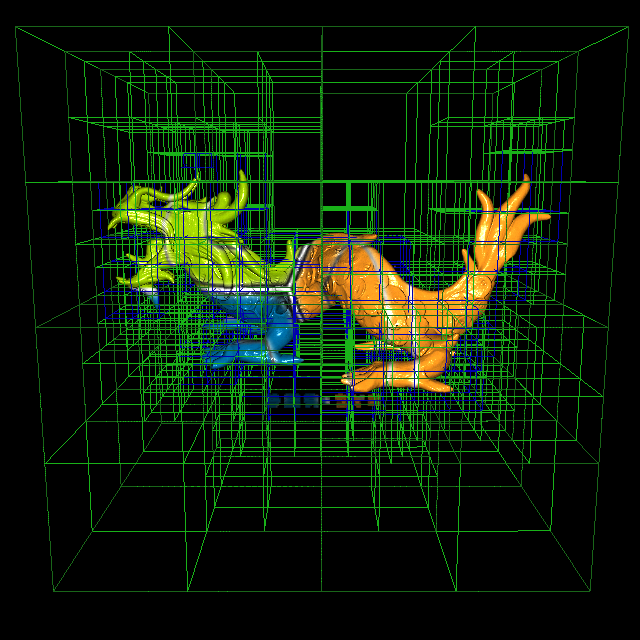source: Cyril Crassin

### SVO - Sparse Voxel Octrees

SVOs:

• Huge Octree (GB's) on HD, load needed parts into memory
• Hybrid approach:
• Primary rays (cam): push geometry through rasterizer
• Following rays: Cone trace through SVO
Cone tracing: inexact, but not noisy $\rightarrow$ perfect for lighting!

Voxel cone tracing:

• Progressively increase step size
• Accumulate light with occlusion
• Stop if occluded or too far
• Bonus: smooth occluders are further away $\rightarrow$ cheaper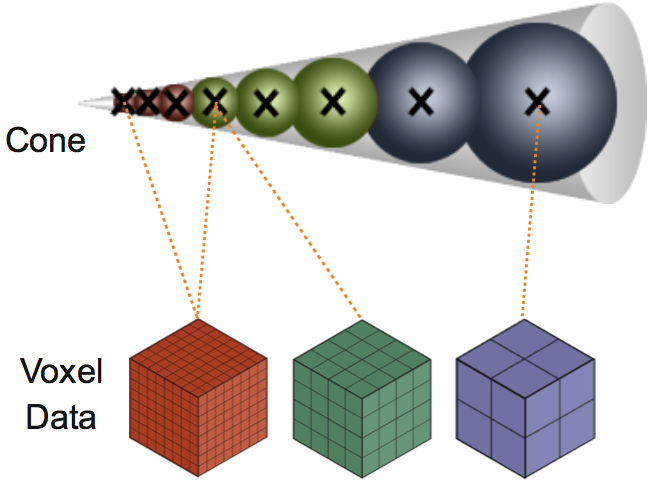source: Epic Games

### SVO - Sparse Voxel Octrees

How do we use the SVO?

• Store distances in the octree
• Store material information

How do we store things in there?
How does the geometry come into play?

• Voxelize the scene into the octree!
• At runtime

... and no, I'm not kidding....

### Unreal Engine 4source: www.geforce.com

### UE4 - Voxel Lighting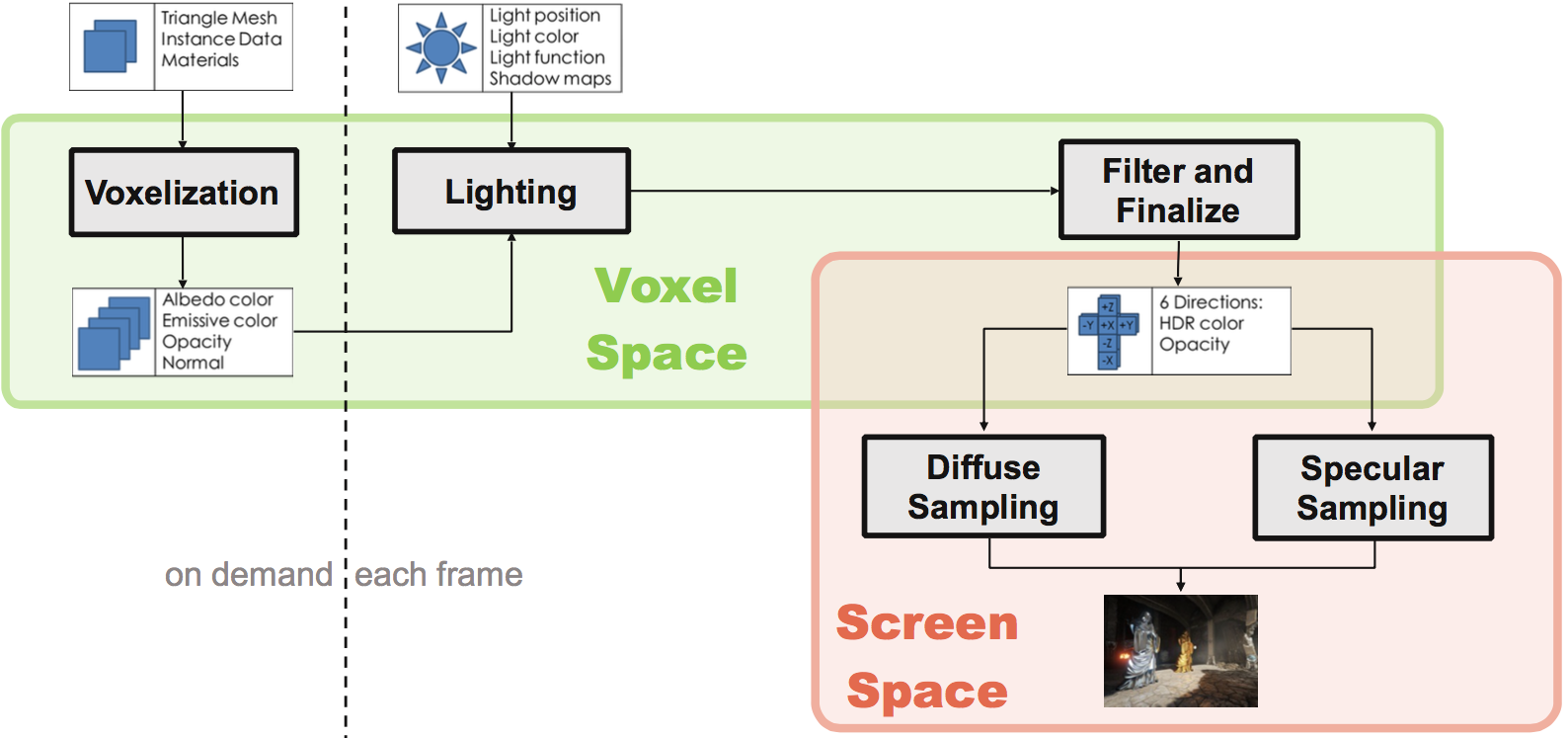source: Epic Games

### UE4 - Voxel Lighting

 Voxel Lighting Data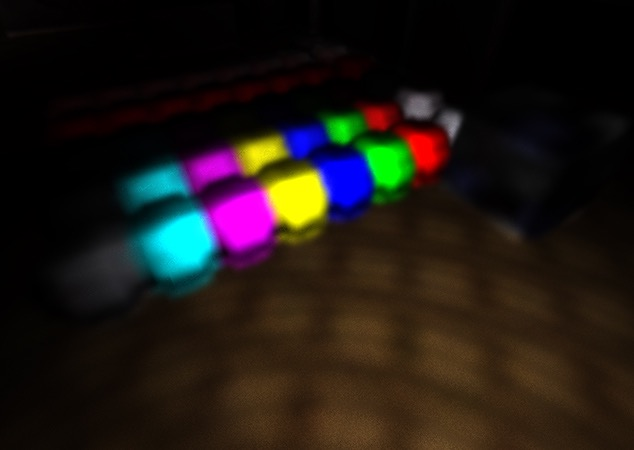Full 3D-Scene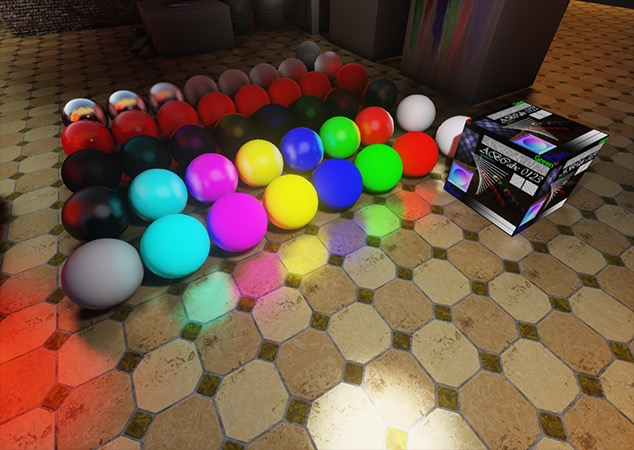source: Epic Games